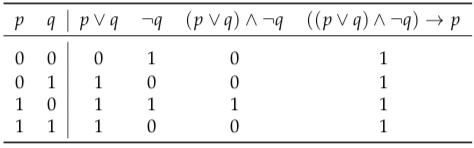# 2.1.J Classifying propositions

Certain types of proposition will play a special role in our further work with logic. In particular, we define tautologies, contradictions, and contingencies as follows:

Definition 2.4.

A compound proposition is said to be a tautology if and only if it is true for all possible combinations of truth values of the propositional variables which it contains. A compound proposition is said to be a contradiction if and only if it is false for all possible combinations of truth values of the propositional variables which it contains. A compound proposition is said to be a contingency if and only if it is neither a tautology nor a contradiction.

For example, the proposition ((∨ q) ∧ ¬q) → is a tautology. This can be checked 2with a truth table:The fact that all entries in the last column are true tells us that this expression is a tautology. Note that for any compound proposition Pis a tautology if and only if ¬Pis a contradiction. (Here and in the future, I use uppercase letters to represent compound propositions. stands for any formula made up of simple propositions, propositional variables, and logical operators.)

Logical equivalence can be defined in terms of tautology:

Definition2.5.

Two compound propositions, and Q, are said to be logically equivalent if and only if the proposition ↔ is a tautology.

The assertion that is logically equivalent to will be expressed symbolically as “≡ Q”. For example, (→ q) ≡ (¬∨ q), and ⊕ ≡ (∨ q) ∧ ¬(∧ q).

What if → and is false? From a false premise we can derive any conclusion (check the truth table of →). So if stands for “I’m the King of the Netherlands”, then → is true for any compound proposition Q. You can substitute anything for Q, and the implication → will hold. For example, it a logically valid deduction that: If I’m the King of the Netherlands, then unicorns exist. Taking this further, from a contradiction we can derive any conclusion. This is called the Principle of Explosion.

Exercises

1. Give the three truth tables that define the logical operators ∧, ∨, and ¬.

2. Some of the following compound propositions are tautologies, some are contradictions, and some are neither (i.e.,, so are contingencies). In each case, use a truth table to decide to which of these categories the proposition belongs:

a) (∧ (→ q)) → q    b) ((→ q) ∧ (→ r)) → (→ r)

c) ∧ ¬p    d) (∨ q) → (∧ q)
e) ∨ ¬p    f) (∧ q) → (∨ q)

3. Use truth tables to show that each of the following propositions is logically equivalent to ↔ q.

a) (→ q) ∧ (→ p)     b) ¬↔ ¬q
c) (→ q) ∧ (¬→ ¬q)     d) ¬(⊕ q)

4. Is → an associative operation? This is, is (→ q) → logically equivalent to → (→ r)? Is↔ associative?

1. Let represent the proposition “You leave” and let represent the proposition “I leave”. Ex- press the following sentences as compound propositions using and q, and show that they are logically equivalent:

a) Either you leave or I do. (Or both!)    b) If you don’t leave, I will.

2. Suppose that represents the proposition “The Earth moves”, represents “The Earth is the centre of the universe”, and represents “Galileo was falsely accused”. Translate each of the following compound propositions into English:

a) ¬∧ c     b) → ¬c

c) ↔ ¬c     d) (→ g) ∧ (→ ¬g)

7. Give the converse and the contrapositive of each of the following English sentences:

a) If you are good, Sinterklaas brings you toys.

b) If the package weighs more than one kilo, then you need extra postage.
c) If I have a choice, I don’t eat courgette.

8. In an ordinary deck of fifty-two playing cards, for how many cards is it truea) that “This card is a ten and this card is a heart”?

b) that “This card is a ten or this card is a heart”?
c) that “If this card is a ten, then this card is a heart”?

d) that “This card is a ten if and only if this card is a heart”?

9.Define a logical operator ↓ so that ↓ is logically equivalent to ¬(∨ q). (This operator is usually referred to as ‘nor’, short for ‘not or’.) Show that each of the propositions ¬p∧ q,∨ q→ q↔ q, and ⊕ can be rewritten as a logically equivalent proposition that uses↓ as its only operator.

10. For our proof that {¬, ∨} is functionally complete, we need to show that all formulas in pro- positional logic can be expressed in an equivalent form using only {¬, ∧, ∨, →, ↔}.

a)  How many unique truth tables exist for formulas containing two atoms?

b)  Create a function for each of the possible truth tables that uses only the 5 operators listed

above.

c)  Give an (informal) argument why this means all formulas in propositional logic can be

expressed using only these five operators.